# 2nd PUC Statistics Question Bank Chapter 7 Statistical Quality control

## Karnataka 2nd PUC Statistics Question Bank Chapter 7 Statistical Quality Control

### 2nd PUC Statistical Quality Control One and Two Marks Questions With Answers

Question 1.
What do you mean by Statistical Quality Control?
The control of quality of manufactured articles using statistical, techniques is called SQC.

Question 2.
Mention two types of causes for variation in a manufacturing process.
Chance causes and Assignable causes.

Question 3.
In X̄ – chart, if one of the sample means lies outside the control lines, what would you conclude?
We conclude that the production process is out of control’ or lack of control.

Question 4.
SQC helps in which type of variation?/ Which type of ’cause of variation’ is detected by SQC.
SQC helps in detecting Assignable causes.

Question 5.
Which cause of variation cannot be eliminated from the process control?
Chance cause of variation.Question 6.
Distinguish between a defect and a defective/what are Defects and Defectives?

• A defect is a quality characteristic, which does not conform to specifications.
• The presence of one or more defects in a product/article is a defective.

Question 7.
Write a merit of ‘Acceptance sampling in “SQC”
It is useful when the items are of destructive in nature.

Question 8.
Give an example for a defect & defective
Misprints, damages in weaving on a cloth, a glass bottle with one or more bubble, excessive thickness of a glass pane, are all defects The cloth, glass bottle, glass pane are all defectives.

Question 9.
Name the Control Chart for variable
X̄ and R charts are used for the control of variables.

Question 10.
Name the control chart used in case of defective in S.Q.C.
np/d-chart

Question 11.
What are process control and product control?.

• Controlling the quality of the product during the manufacturing process itself is called process control.
• Controlling the quality of the finished products/manufactured products is called product control.

Question 12.
Mention any two uses/advantages of SQC

• It improve the quality and to decrease the proportion of defectives
• It provides greater quality assurance at lower inspection cost.

Question 13.
What are control charts/What is a control chart?.
Control chart is graphical device, which used to verify whether the production process is in Statistical control or not.

Question 14.
What is Acceptance-sampling / Acceptance sampling plan?
The process of inspection of manufactured lot for acceptability is called Acceptance sampling plan

Question 15.
What do you mean by Single and Double Sampling Plans?
In a single sampling plan, the decision about accepting or rejecting a lot is based on one sample only. Where as in a double sampling plan, the decision is based on two samples drawn from a lot.Question 16.
Write Upper and Lower control Limits for ‘number of defects’ chart when standards are given.
Number of defects use C-chart: U.C.L = $$\lambda^{\prime}+3 \sqrt{\lambda^{\prime}}$$ L.C.L = $$\lambda^{1}-3 \sqrt{\lambda^{1}}$$

Question 17.
If c̅ = 2 find U.C.L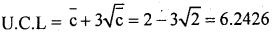Question 18.
If p’ = 0.13 and n = 100, find the value of central line(CL) for np-chart.
C.L = np’ = 100 × 0.13 = 13

Question 19.
If p’ = 0.02 and n = 100, calculate U.C.L for d – chart (np – chart).
np’ + $$3 \sqrt{n P^{\prime} Q^{\prime}}$$ =100 × 0.02 + $$3 \sqrt{100 \times 0.02 \times 0.98}$$ = 3.4

Question 20.
What do you mean by statistical quality control?
The control of quality of manufactured products using statistical techniques is called statistical quality control.

Question 21.
What are chance causes?
A small amount of variation in the quality of the product for no specific cause/reason, and such variation is called chance causes.

Question 22.
What are assignable causes?
Variation in the quality of the product due to use of substandard raw material, improper machine setting, wrong handling of machines, power variation etc., and such variations are called assignable causes.

Question 23.
What is a control chart?
Control chart is a graphical device which is used to verify whether the production process is in statistical control or not.

Question 24.
Name the control chart used in case of defects in statistical quality control.
C-chart.

Question 25.
Write down control limits for x-charts when standards are given and not given.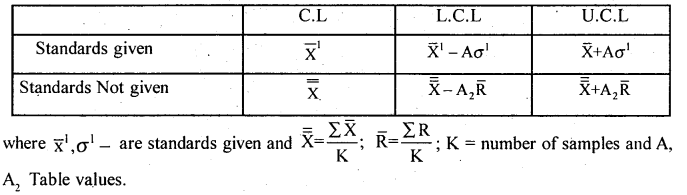Question 26.
Mention any two differences between single sampling plan and double sampling plan.
In single sampling plan, decision about accepting or rejecting a lot is based on one sample only, whereas in double sampling plan decision is based on two samples.
In S.S.P. the lot is accepted if:
d ≤ c , where d = no. of defectives, c-acceptance number and

In D.S.P the lot is accepted if:
d1 ≤ c2 and (d1 + d2) ≤ c2 .
where d1,d2 – no. of defectives ; c1, c2 – acceptance number .

Question 27.
Write down control limits for R-charts when standards are given and not given. Ans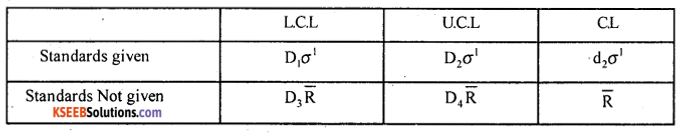Where σ’ – Standard given and $$\overline{\mathrm{R}}=\frac{\Sigma \mathrm{R}}{\mathrm{K}}$$ ; and D1 D2, D3 and D4 table constants.

Question 28.
Write down control limits for number of defectives (np/d -chart) when standards given and not given.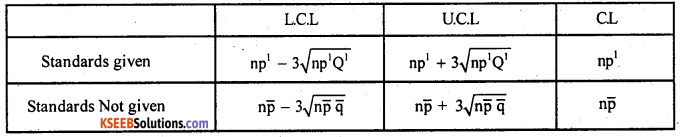Write P’ – given standard, $$\overline{\mathrm{P}}=\frac{\Sigma d}{\mathrm{nK}}$$ ; d – number of defectives, K – number of samples n – sample size

Question 29.
Write down control limits for number of defects (C – chart)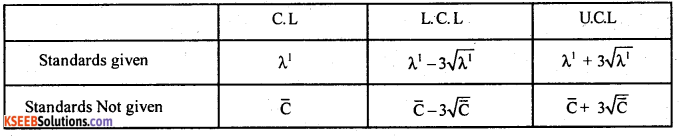Where λ’ – given standard, $$\overline{\mathrm{C}}=\frac{\Sigma \mathrm{C}}{\mathrm{K}}$$ , and C – number of defects,
K – number of samples

Question 30.
Give two merits and demerits of single sampling plan and double sampling plan.
(a) Decision and testing procedure of single sampling plan is easier than double sampling plan.
(b) Decision based on two samples is more reliable and has psychological advantage of giving the lot a second chance compared to single sampling plan.

Question 31.
Advatage: When the items are of distructive in nature, it is less espensive, requires few staff as compared to 100% inspection.
Disadvantages: There is a risk of accepting bad lot and rejecting good lot. Moreover double sampling plans more costly and time consuming.

Question 32.
If P| = 0.02 and n = 100, calculate the upper control limit for d-chart (np-chart).
Answer: U.C.L when standards given is :
U.C.L = np’+$$3 \sqrt{n p^{\prime} Q^{\prime}}$$ = 100 × 0.02 + $$3 \sqrt{100 \times 0.02 \times 0.98}$$ = 2 + $$3 \sqrt{1.96}$$
U.C.L = 6.2

### 2nd PUC Statistical Quality Control Five Marks Questions With Answers

Question 1.
Measurements in mean (X̄) and Range (R) from 10 samples each of size 5 gave results as X̿ = 80, R̄=3.5 Find 3σ conttrol limits for drawing X̄ chart ( A2  is given as 0.557)
Given : X̿ = 80, R̄ =3.5 n = 5
The control limits for X̄ chart are (standards are not given)
C.L = X̿ = 80
L.C.L = X̿ – A2R̄ =80-0.557 × 3.5 = 78.05
U.C.L = X̿ + A2R̄= 80 + 0.557 × 3.5 = 81.95

Question 2.
A drill drills holes with standard deviation of depth 0.05 cms and mean depth of holes is 5.70cms. Find 3 σ control limits for mean of samples of size 4. Given A = 1.500.
Given: X̄1 =5.70 cms : σ1 =0.05 cms, n=4
The 3σ – control limits for X̄ – chart (standards are given) are
C.L = X̄1 = 5.70
L.C.L = X̄1 – Aσ1 =5.70 – 1,5 × 0.05 = 5.625
U.C.L = X̄1 + Aσ1 =5-70 + 1.5 × 0.05 = 5.775

Question 3.
If X̄1 = 35.5 , σ1 = 3.5 and n = 5. Write down the control limits for X̄ and R – charts.
Given: X̄1 = 35.5, σ1=3.5, n = 5, A = 1.342 [From the table]
The control limits for X̄ – chart (standards are given) are:
C.L = X̄1 = 35.5
L.C.L = X̄1 – Aσ1 = 35.5 – 1.342 × 3.5 = 30.803
U.C.L = X̄1 + Aσ1 = 35.5 + 1.342 × 3.5 = 40.197
The control limits for R – chart are (standards are given) : d2 = 2.326, D1 = 0, D2 = 4.918 (From the table)
C.L = d2 σ1 = 2.326 × 3.5 = 8.141
L.C.L = D1 σ1 = 0 × 3.5 = 0 .
U.C.L = D2σ1 = 4.918 × 3.5 = 17.213

Question 4.
If X̿ = 112.6, R̄ = 4.2, n = 4, write down the control limits for X̄ and R- charts.
Given X̿ = 112.6, R̄ = 4.2, n = 4, and the constants from the table A2 = 0.729, D3 = 0, D4= 2.115
When standards are not given:
The control limits for R- chart are:
C.L = X̿ = 112.6
L.C.L = X̿ – A2R̄ =112.6 – 0.729 × 4.2 = 109.54
U.C.L = X̿ + A2R̄ = 112.6 + 0.729 × 4.2= 115.66
The control limits for R – chart are :
C.L = R̄ = 4.2
L.C.L =D3R̄ = 0 × 4.2 = 0
U.C.L = D4R̄ = 2.115 × 4.2 = 8.883Question 5.
Given fraction defective = 0.05, and sample size 8. Write down the control limits for number of defectives
Given: P1 = 0.05, n = 8,
Here Q1 = 1 – P1 = 1 – 0.05 = 0.95
The control limits for number of defectives np/d – chart (When standards are given) are:
C.L = np1 =8 × 0.05 = 0.4
L.C.L = np1 – $$3 \sqrt{n p^{\prime} Q^{\prime}}$$ = 8 × 0.05 – $$3 \sqrt{8 \times 0.5 \times 0.05}$$ =-1.45 = 0 (Taken as 0)
U.C.L = np1 + $$3 \sqrt{n p^{\prime} Q^{\prime}}$$ = 8 × 0.05 + $$3 \sqrt{8 \times 0.5 \times 0.05}$$ = 2.25

Question 6.
In a bulb manufacturing company, the proportion of defectives is 0.08. If the process control is based on samples of size 150 each. Write down the control limit for np or d-chart.
Given: P1 = 0.08, n = 150
(∴ Q1= 1 -P1)= 1 – 0.08 = 0.92
The control limits for np – chart are
C.L =np1= 150 × 0.08 = 12
L.C.L = np1 – $$3 \sqrt{n p^{\prime} Q^{\prime}}$$= 150 × 0.08 – $$3 \sqrt{150 \times 0.08 \times 0.92}$$ x 0.92 = 2.032
U.C.L = np1 + $$3 \sqrt{n p^{\prime} Q^{\prime}}$$ =150 × 0.08 + $$3 \sqrt{150 \times 0.08 \times 0.92}$$ =21.96 = 22

Question 7.
The following data show the values of sample mean (X̄) and range (R) for samples of size 5 each. Calculate values for central line and control limits for mean chart. (Given A2 = 0.577 for n = 5)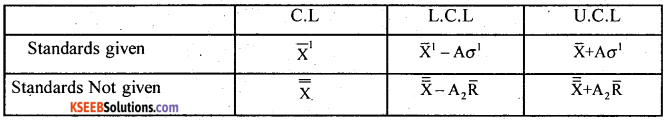Here Standards are not given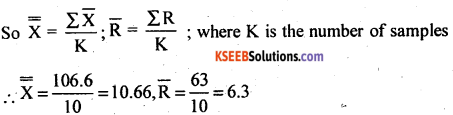The control limits for X̄ – chart are :
C.L = X̿ = 10.66
L.C.L = X̿ – A2R̄= 10.66 – 0.577 × 6.3 = 7.025
U.C.L = X̿ + A2R̄ = 10.66 + 0.577 × 6.3 = 14.30

Question 8.
You are given values of samples means (X̄) and Range (R) for 6 samples of the size 4 each. Find control limits for mean and Range charts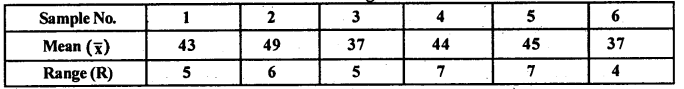you may use the following control chart constants
For n = 4, A2 = 0.729, D3 = 0; D4 = 2.282
Here standards are not given and K = 4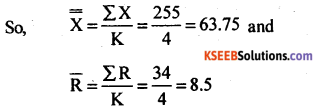The control limits for X – chart are :
C.L = X̿ = 63.75
L.C.L = X̿ – A2R̄ = 63.75 – 0.729 × 8.5 = 57.55
U.C.L = X̿ + A2R̄ = 63.75 + 0.729 × 8.5 = 69.55
The control limits for R – chart are:
C.L = R̄ = 8.5
L.C.L = D3R̄ = 0 × 8.5 = 0
U.C.L = D4R̄ =2.282 × 8.5 = 19.40

Question 9.
The following are the number of defects noted in the final inspection of balls of woolen cloth 0, 3, 1, 4, 2, 2, 1, 3, 5, 0, 2, 1, 0, 3.
Write down an appropriate control limits
Here Standards are not given and number of defects are given.
The chart would be C-chart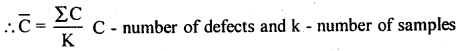C̅ = $$\frac { 27 }{ 15 }$$ = 1.8 15
C.L = C̅ = 1.8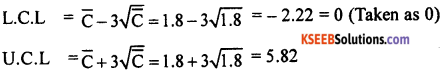Question 10.
If λ1 = 2.5 Write down the control limits for C-Chart.
Answer: Given Standard is : λ1 = 2.5
The control limits for c-chart are :
C.L = λ1 =2.5
L.C.L = $$\lambda^{1}-3 \sqrt{\lambda^{1}}=2.5-3 \sqrt{2.5}$$ = -2.24 = 0 (Taken as ‘o’. )
U.C.L = $$\lambda^{1}+3 \sqrt{\lambda^{1}}=2.5-3 \sqrt{2.5}$$= 7.24Question 11.
In a printing industry, at regular intervals, cloth is inspected for defects in printing. If on an average 0.5 defects are expected per square metre, obtain suitable control limits.
Given : Standard λ1 = 0.5
The control limits for C-chart are :
C.L = λ1 = 0.5
L.C.L = $$\lambda^{\prime}-3 \sqrt{\lambda^{\prime}}=0.5-3 \sqrt{0.5}$$ = -1.62 =0 (Taken as 0)
U.C.L = $$\lambda^{\prime}-3 \sqrt{\lambda^{\prime}}=0.5+3 \sqrt{0.5}$$ =2.62

Question 12.
From a soap manufacturing machine, samples consisting of 5 packed soap cakes are drawn and the following observations were recorded: X̄1 = 75 gms ; σ1 = 3 gms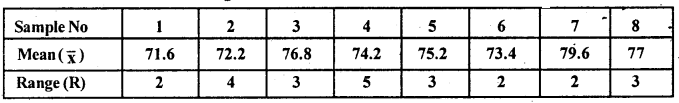draw X̄-chart and comment
Given: n – 5, X̄1 = 75 gms , σ1 = 3 gms (Standards are given)
The X̄ – control limits are
C.L = X̄1 = 75
L C.L = X̄1 – Aσ1 = 75 – 1.342 × 3S = 70.97 = 71
U.C.L = X̄1 + Aσ1 = 75 + 1.342 × 3 = 79.03 = 79
The X̄ – chart and the plotted points are as shown below: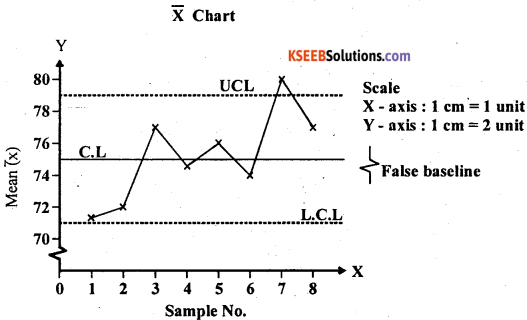Interpretation: In X̄ -chart one of the point lie outside the UCL, the process is out of control.

Question 13.
If the standards are known to be X̄1 =20 and σ1 = 6, construct X̄ chart for the data sample size n = 5 and A = 1.342)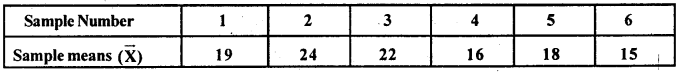Given :n = 5, A = 1.342, X̄1 =20, σ1 =6
Standards are given
The control limits for X̄ chart are:
C.L = X̄1 = 20
L.C.L = X̄1 – Aσ1 =20 – 1.342 × 6 = 11.948 = 11.95
U.C.L. = X̄1 + Aσ1 =20 + 1.342 × 6 = 23.052 = 28.05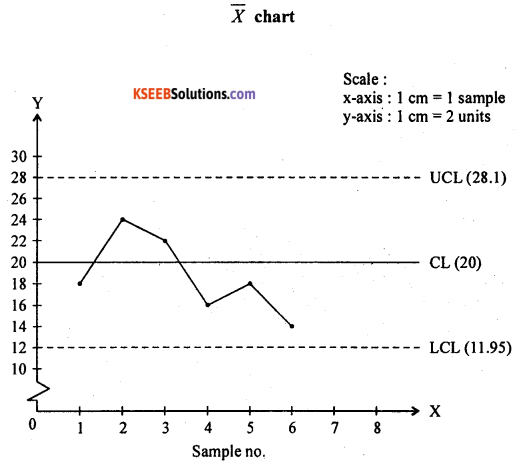Interpretation: All the plotted lie with in the control limits. Therefore the production process is under statistical control.

Question 14.
Construct a suitable control chart for the following data and state your conclusion.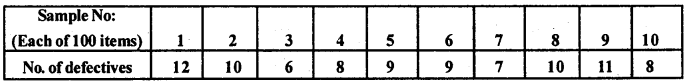Here standards are not given;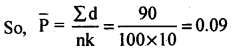∴ q̄ =l – p̄ = 1 – 0.09 = 0.91
where n = sample size, = 100
K = number of samples and d = number of defectives
The control limits for np-chart (d-chart) are:
C.L = np̄ =100 × 0.09 = 9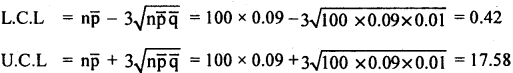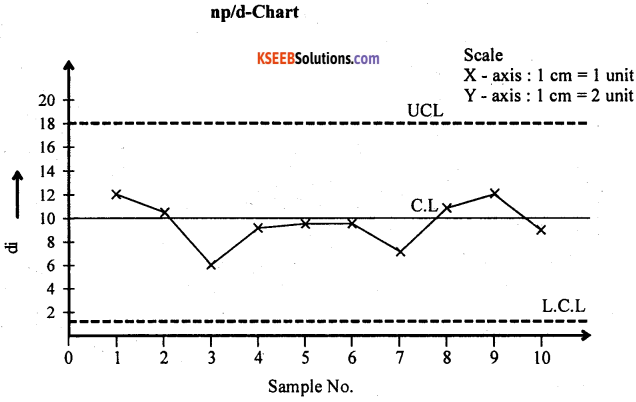Question 15.
During an examination of equal lengths of cloth, the following are the number of defects observed.
2, 3, 4, 0, 5, 6, 7, 4, 3,2.
Draw a control chart for the number of defects and comment whether the process is under control or not
Here Standards are not given and So,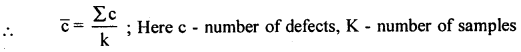c̄ = $$\frac { 36 }{ 10 }$$ = 3.6
The control limits for c-chart are
C.L = c̄ =3.6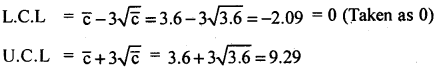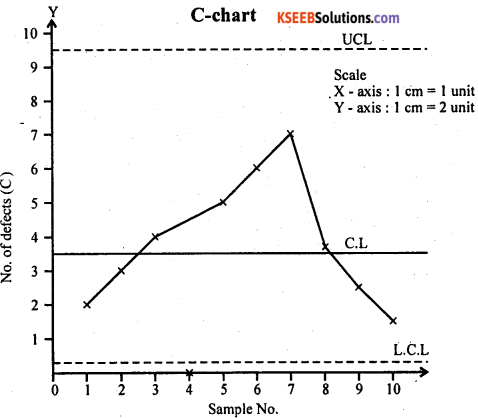Interpretation : The points are with in central limits, the process is under control

Question 16.
Given D3 = 0, D4, =2.115, and R̄ = 4
draw R-chart for the following data.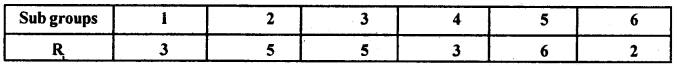The control limits for R-chart are :
when standards are not given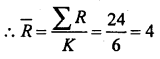k = number of samples = 6
C.L. = R̄ = 4
L.C.L = D3R̄ = 0 × 4 = 0
U. C. L = D4R̄ = 2.115 × 4 = 8.46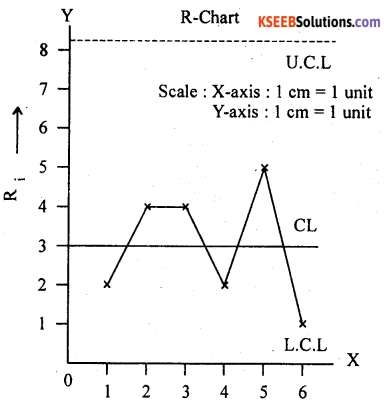Interpretation : All points are with in control limits. The process is under control

Question 17.
A company manufactures flooring titles. samples of 100 tiles are drawn at regular intervals. The number of defective tiles are given below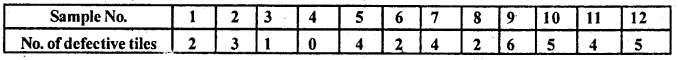Find suitable control limits for the above data.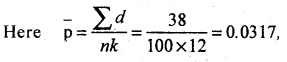L.C.L = $$n \bar{p}-3 \sqrt{n \overline{p q}}$$
= 100 × 0.0317 – $$3 \sqrt{100 \times 0.0137 \times 0.9683}$$= -2.09 (Taken as 0)
U.C.L = $$n \bar{p}+3 \sqrt{n \overline{p q}}$$= 100 x 0.0317 – $$3 \sqrt{100 \times 0.0137 \times 0.9683}$$